Test: Asymptotic Worst Case Time & Space Complexity- 4

# Test: Asymptotic Worst Case Time & Space Complexity- 4 - Computer Science Engineering (CSE)

Test Description

## 20 Questions MCQ Test GATE Computer Science Engineering(CSE) 2024 Mock Test Series - Test: Asymptotic Worst Case Time & Space Complexity- 4

Test: Asymptotic Worst Case Time & Space Complexity- 4 for Computer Science Engineering (CSE) 2023 is part of GATE Computer Science Engineering(CSE) 2024 Mock Test Series preparation. The Test: Asymptotic Worst Case Time & Space Complexity- 4 questions and answers have been prepared according to the Computer Science Engineering (CSE) exam syllabus.The Test: Asymptotic Worst Case Time & Space Complexity- 4 MCQs are made for Computer Science Engineering (CSE) 2023 Exam. Find important definitions, questions, notes, meanings, examples, exercises, MCQs and online tests for Test: Asymptotic Worst Case Time & Space Complexity- 4 below.
Solutions of Test: Asymptotic Worst Case Time & Space Complexity- 4 questions in English are available as part of our GATE Computer Science Engineering(CSE) 2024 Mock Test Series for Computer Science Engineering (CSE) & Test: Asymptotic Worst Case Time & Space Complexity- 4 solutions in Hindi for GATE Computer Science Engineering(CSE) 2024 Mock Test Series course. Download more important topics, notes, lectures and mock test series for Computer Science Engineering (CSE) Exam by signing up for free. Attempt Test: Asymptotic Worst Case Time & Space Complexity- 4 | 20 questions in 60 minutes | Mock test for Computer Science Engineering (CSE) preparation | Free important questions MCQ to study GATE Computer Science Engineering(CSE) 2024 Mock Test Series for Computer Science Engineering (CSE) Exam | Download free PDF with solutions
 1 Crore+ students have signed up on EduRev. Have you?
Test: Asymptotic Worst Case Time & Space Complexity- 4 - Question 1

### Which of the following is TRUE?

Detailed Solution for Test: Asymptotic Worst Case Time & Space Complexity- 4 - Question 1

AVL tree is a balanced tree.
AVL tree's time complexity of searching = θ(logn)
But a binary search tree, may be skewed tree, so in worst case BST searching time = θ(n)

Test: Asymptotic Worst Case Time & Space Complexity- 4 - Question 2

### The two numbers given below are multiplied using the Booth's algorithm. Multiplicand : 0101 1010 1110 1110 Multiplier: 0111 0111 1011 1101 How many additions/Subtractions are required for the multiplication of the above two numbers?

Test: Asymptotic Worst Case Time & Space Complexity- 4 - Question 3

### If we use Radix Sort to sort n integers in the range (nk/2,nk], for some k>0 which is independent of n, the time taken would be?

Detailed Solution for Test: Asymptotic Worst Case Time & Space Complexity- 4 - Question 3

Radix sort time complexity = O(wn)
for n keys of word size= w
=>w = log(nk
O(wn)=O(klogn.n)
=> kO(nlogn)

Test: Asymptotic Worst Case Time & Space Complexity- 4 - Question 4

The auxiliary space of insertion sort is O(1), what does O(1) mean?

Detailed Solution for Test: Asymptotic Worst Case Time & Space Complexity- 4 - Question 4

The term O(1) states that the space required by the insertion sort is constant i.e., space required doesn't depend on input.

Test: Asymptotic Worst Case Time & Space Complexity- 4 - Question 5

What is the value of following recurrence.

T(n) = T(n/4) + T(n/2) + cn^2
T(1) = c
T(0) = 0

Q. Where c is a positive constant

Detailed Solution for Test: Asymptotic Worst Case Time & Space Complexity- 4 - Question 5

Following is the initial recursion tree for the given recurrence relation.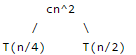If we further break down the expression T(n/4) and T(n/2), we get following recursion tree.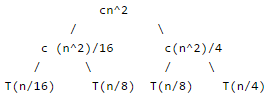Breaking down further gives us following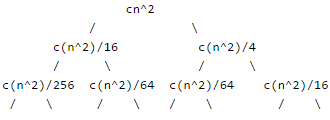To know the value of T(n), we need to calculate sum of tree nodes level by level. If we sum the above tree level by level, we get the following series T(n) = c(n^2 + 5(n^2)/16 + 25(n^2)/256) + .... The above series is geometrical progression with ratio 5/16 To get an upper bound, we can sum the above series for infinite terms. We get the sum as (n^2) / (1 - 5/16) which is O(n^2)

Test: Asymptotic Worst Case Time & Space Complexity- 4 - Question 6

What is the value of following recurrence. T(n) = 5T(n/5) +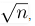, T(1) = 1, T(0) = 0

Detailed Solution for Test: Asymptotic Worst Case Time & Space Complexity- 4 - Question 6

The given solution can be solved using Master Method. It falls in Case 1.

Test: Asymptotic Worst Case Time & Space Complexity- 4 - Question 7

What is the worst case time complexity of following implementation of subset sum problem.

// Returns true if there is a subset of set[] with sun equal to given sum

bool isSubsetSum(int set[], int n, int sum)

{

// Base Cases

if (sum == 0)

return true;

if (n == 0 && sum != 0)

return false;

// If last element is greater than sum, then ignore it

if (set[n-1] > sum)

return isSubsetSum(set, n-1, sum);

/* else, check if sum can be obtained by any of the following

(a) including the last element

(b) excluding the last element   */

return isSubsetSum(set, n-1, sum) ||

isSubsetSum(set, n-1, sum-set[n-1]);

}

Detailed Solution for Test: Asymptotic Worst Case Time & Space Complexity- 4 - Question 7

Following is the recurrence for given implementation of subset sum problem T(n) = 2T(n-1) + C1 T(0) = C1 Where C1 and C2 are some machine specific constants. The solution of recurrence is O(2^n) We can see it with the help of recurrence tree method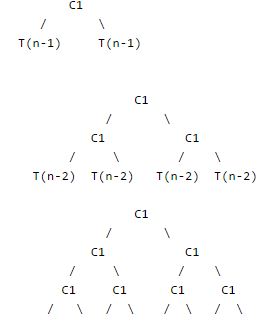If we sum the above tree level by level, we get the following series T(n) = C1 + 2C1 + 4C1 + 8C1 + ...
The above series is Geometrical progression and there will be n terms in it.
So T(n) = O(2^n)

Test: Asymptotic Worst Case Time & Space Complexity- 4 - Question 8

Suppose T(n) = 2T(n/2) + n, T(0) = T(1) = 1 Which one of the following is false.
a) T(n) = O(n^2)
b) T(n) = θ(nLogn)
c) T(n) = Ω(n^2)
d) T(n) = O(nLogn)

Test: Asymptotic Worst Case Time & Space Complexity- 4 - Question 9

Consider the following recurrence: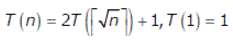Q. Which one of the following is true?

Detailed Solution for Test: Asymptotic Worst Case Time & Space Complexity- 4 - Question 9

This question can be solved by first change of variable and then Master Method.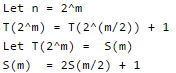Above expression is a binary tree traversal recursion whose time complexity is θ(m). You can also prove using Master theorem.

S(m) = θ (m)
= θ (log n)

/* since n = 2^m */

Now, let us go back to the original recursive function T(n)

T(n) = T(2^m) = s(m)

=  θ (log n)

Test: Asymptotic Worst Case Time & Space Complexity- 4 - Question 10

The running time of an algorithm is represented by the following recurrence relation: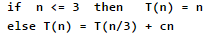Q. Which one of the following represents the time complexity of the algorithm?

Detailed Solution for Test: Asymptotic Worst Case Time & Space Complexity- 4 - Question 10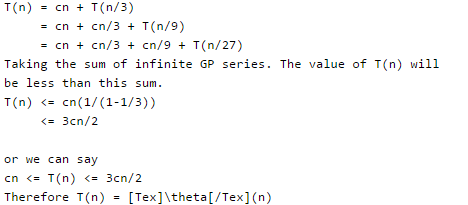This can also be solved using Master Theorem for solving recurrences. The given expression lies in Case 3 of the theorem.

Test: Asymptotic Worst Case Time & Space Complexity- 4 - Question 11

The running time of the following algorithm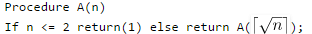is best described by

Test: Asymptotic Worst Case Time & Space Complexity- 4 - Question 12

What is the time complexity of the following recursive function: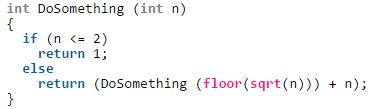Detailed Solution for Test: Asymptotic Worst Case Time & Space Complexity- 4 - Question 12

Recursive relation for the DoSomething() is

T(n) = T(√n) + C1 if n > 2

We have ignored the floor() part as it doesn't matter here if it's a floor or ceiling.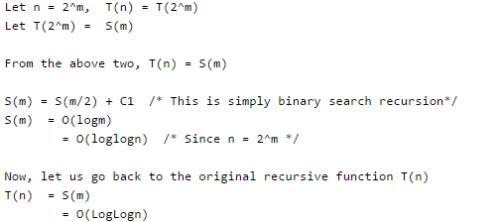Test: Asymptotic Worst Case Time & Space Complexity- 4 - Question 13

The time complexity of the following C function is (assume n > 0

int recursive (int n)
{
if(n == 1)
return (1);
else
return (recursive (n-1) + recursive (n-1));
}

Detailed Solution for Test: Asymptotic Worst Case Time & Space Complexity- 4 - Question 13

Recursive expression for the above program will be.

T(n) = 2T(n-1) + c
T(1) = c1.

Let us solve it.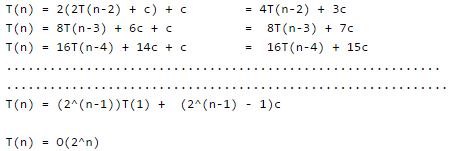Test: Asymptotic Worst Case Time & Space Complexity- 4 - Question 14

Consider the following recurrence T(n) = 3T(n/5) + lgn * lgn What is the value of T(n)?

Detailed Solution for Test: Asymptotic Worst Case Time & Space Complexity- 4 - Question 14

By Case 1 of the Master Method, we have T(n) = θ(n ^ (log5(3))). [^ is for power]

Test: Asymptotic Worst Case Time & Space Complexity- 4 - Question 15

Consider the following recurrence.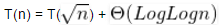Q. What is the value of recurrence?

Detailed Solution for Test: Asymptotic Worst Case Time & Space Complexity- 4 - Question 15

Change of variables: let m = lg n. Recurrence becomes S(m) = S(m/2) + θ(lgm). Case 2 of master’s theorem applies, so T(n) = θ( (log Log n) ^ 2).

Test: Asymptotic Worst Case Time & Space Complexity- 4 - Question 16

Which one of the following correctly determines the solution of the recurrence relation with T(1) = 1?

T(n) = 2T(n/2) + Logn

Detailed Solution for Test: Asymptotic Worst Case Time & Space Complexity- 4 - Question 16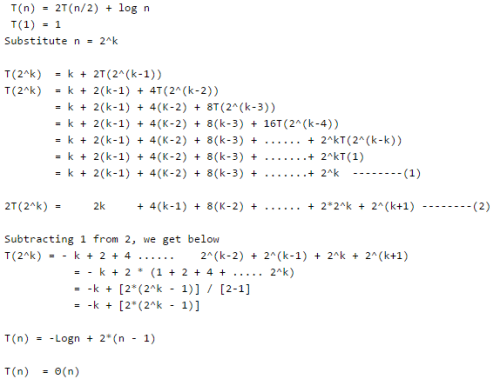Test: Asymptotic Worst Case Time & Space Complexity- 4 - Question 17

Consider the following recurrence relation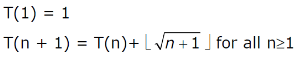The value of T(m2) for m ≥ 1 is

Detailed Solution for Test: Asymptotic Worst Case Time & Space Complexity- 4 - Question 17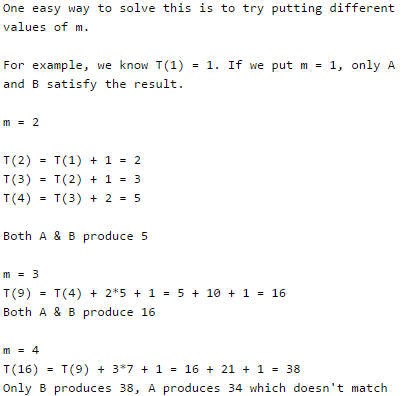Test: Asymptotic Worst Case Time & Space Complexity- 4 - Question 18

The solution to the recurrence equation T(2k) = 3 T(2k-1) + 1, T (1) = 1, is:

Detailed Solution for Test: Asymptotic Worst Case Time & Space Complexity- 4 - Question 18

We have T (2k) = 3  T (2k-1) + 1 = 32  T (2k-2) + 1 + 3 = 33  T (2k-3) + 1 + 3 + 9 . . . (k steps of recursion (recursion depth)) = 3k  T (2k-k) + (1 + 3 + 9 + 27 + ... + 3k-1) = 3k + (( 3k - 1)/2) = ((2 * 3k) + 3k - 1)/2 = ((3 * 3k) - 1)/2 = (3k+1 - 1)/2 Hence, B is the correct choice.

Test: Asymptotic Worst Case Time & Space Complexity- 4 - Question 19

Select the correct asymptotic complexity of an algorithm with runtime T(n, n) where

T(x, c) = Θ(x) for c <= 2,
T(c, y) = Θ(y) for c <= 2, and
T(x, y) = Θ(x+y) + T(x/2, y/2)

Detailed Solution for Test: Asymptotic Worst Case Time & Space Complexity- 4 - Question 19

The recurrence is

T(x, y) = Θ(x+y) + T(x/2, y/2)

It can be written as below.

T(x, y) = Θ(x+y) + Θ((x+y)/2) + Θ((x+y)/4) + Θ((x+y)/8) .....
T(x, y) = Θ((x+y) + (x+y)/2 + (x+y)/4 + (x+y)/8 ..... )

The above expression forms a geometric series with ratio as 2 and starting element as (x+y)/2 T(x, y) is upper bounded by Θ(x+y) as sum of infinite series is 2(x+y). It is lower bounded by (x+y)

Test: Asymptotic Worst Case Time & Space Complexity- 4 - Question 20

Let f(n) = n and g(n) = n(1+sin n), where n is a positive integer. Which of the following statements is/are correct?

I. f(n) = O(g(n))
II. f(n) = Ω(g(n))

Detailed Solution for Test: Asymptotic Worst Case Time & Space Complexity- 4 - Question 20

The value of sine function varies from -1 to 1.

For sin = -1 or any other negative value, I becomes false.

For sin = 1 or any other negative value, II becomes false

## GATE Computer Science Engineering(CSE) 2024 Mock Test Series

151 docs|216 tests
Information about Test: Asymptotic Worst Case Time & Space Complexity- 4 Page
In this test you can find the Exam questions for Test: Asymptotic Worst Case Time & Space Complexity- 4 solved & explained in the simplest way possible. Besides giving Questions and answers for Test: Asymptotic Worst Case Time & Space Complexity- 4, EduRev gives you an ample number of Online tests for practice

## GATE Computer Science Engineering(CSE) 2024 Mock Test Series

151 docs|216 tests